## research papersJOURNAL OFSYNCHROTRONRADIATION
ISSN: 1600-5775
Volume 25| Part 4| July 2018| Pages 1135-1143

## Distributed X-ray photon correlation spectroscopy data reduction using Hadoop MapReduce

aX-ray Science Division, Argonne National Laboratory, 9700 South Cass Avenue, Lemont, IL 60439, USA, and bAPS Engineering Support, Argonne National Laboratory, 9700 South Cass Avenue, Lemont, IL 60439, USA
*Correspondence e-mail: fkhan@anl.gov

(Received 20 November 2017; accepted 18 April 2018; online 14 June 2018)

Multi-speckle X-ray photon correlation spectroscopy (XPCS) is a powerful technique for characterizing the dynamic nature of complex materials over a range of time scales. XPCS has been successfully applied to study a wide range of systems. Recent developments in higher-frame-rate detectors, while aiding in the study of faster dynamical processes, creates large amounts of data that require parallel computational techniques to process in near real-time. Here, an implementation of the multi-tau and two-time autocorrelation algorithms using the Hadoop MapReduce framework for distributed computing is presented. The system scales well with regard to the increase in the data size, and has been serving the users of beamline 8-ID-I at the Advanced Photon Source for near real-time autocorrelations for the past five years.

### 1. Introduction

X-ray photon correlation spectroscopy (XPCS) is a powerful technique for characterizing the dynamic nature of complex materials over a wide range of time and length scales. Since the very first demonstration of feasibility of observation of speckles by coherent hard X-rays (Sutton et al., 1991), XPCS has been successfully applied to study a wide range of systems encompassing both soft and hard matter. The studies so far have covered diverse systems, such as colloidal suspensions (Lurio et al., 2000; Sikorski et al., 2011a), gels (Bandyopadhyay et al., 2004; Madsen et al., 2010), liquid crystals (Sikharulidze et al., 2002), polymers (Kim et al., 2003; Jiang et al., 2007), liquid surfaces (Madsen et al., 2004) and hard materials (Fluerasu et al., 2005; Ruta et al., 2014). A variant of the technique, termed X-ray speckle visibility spectroscopy, has also been employed for the measurement of dynamics. In this technique, the X-ray speckle contrast within a single exposure can be related to the relaxation time of the intermediate scattering function (DeCaro et al., 2013; Hruszkewycz et al., 2012; Li et al., 2014) and thus has the ability to measure dynamical time scales faster than the repetition rate of area detectors. The optical analog of XPCS, termed dynamic light scattering, typically used point detectors to measure temporal correlations. On the contrary, because of the low coherent signal levels with X-rays, the XPCS scientific community developed multi-speckle techniques from the beginning by applying area detectors (Lumma et al., 2000). Due to sustained developments in area detector technology from early CCD detectors to fairly parallel readout CCD detectors (Denes et al., 2009) to fully parallel pixel array detectors (Renzi et al., 2002; Broennimann et al., 2006; Pennicard et al., 2013), the data rates have increased by four to five orders of magnitude over the last 15 years with particularly notable increases in the last few years. A recent development in the detector technology is the concept of a vertically integrating detector that has been prototyped and successfully tested for XPCS (Rumaiz et al., 2016).

### 2. Background

The recent development of higher-frame-rate detectors allows the investigation of faster dynamic processes. A consequence of these detector advancements is the creation of large amounts of two-dimensional (2-D) data. It is imperative that the data are processed within the time that it takes to collect the next data so that the user is able to make a judicious selection of measurement conditions. In this paper, we define and refer to this condition as near real-time. Parallel computational techniques and high-performance computing (HPC) resources are thereby an absolute requirement to handle this increase in data volume and rate.

To the best of our knowledge, while the XPCS beamlines at different synchrotron radiation facilities have developed their own data analysis software packages using high-level languages such as MATLAB or Python, the only HPC implementation based on a lower-level programming language that has been reported in the literature has been from the Advanced Photon Source (APS) (Sikorski et al., 2011b; Tieman et al., 2011). Some of the limitations of this previous implementation are that it did not support the computation of sparse data without transforming to a dense format, and it did not scale with the number of frames in a time series.

The single most important parameter that characterizes the dynamic response of a system is the dynamic structure factor S(q,t) or the normalized intermediate structure factor (ISF) g1(q,t) which is related to the experimentally measured and computed intensity–intensity autocorrelation function, g2(q,t) (Berne & Pecora, 2000). A typical XPCS dataset is acquired as a time series of 2-D frames from an area detector operating at a fixed frame spacing as shown schematically in Fig. 1. The analysis of XPCS data is based on computing individual pixel correlations in the time domain, and then averaging such correlations over a group of pixels that constitute a user-specified equivalent wavevector q. The wavevector is inversely related to the length scale in the sample being measured. It can be readily seen that the input time series data in its atomic representation adheres to the form (time, pixel, intensity). This representation naturally fits into the paradigm of MapReduce, which is a well established computing platform that has been proven to be scalable for big data' distributed over hundreds or thousands of servers. We have thereby developed an implementation based on Hadoop MapReduce using two modest distributed computing systems that perform in near real-time for both small and large datasets.Figure 1 Conceptual representation of the time series nature of X-ray data captured by the area detector. Each frame consists of a fixed number of pixels and is separated by a fixed time. The correlation is computed for each pixel in the time domain and then averaged over a group of nominally equivalent pixels.

The dynamics in the sample as a function of scattering wavevector q (inverse length scale) are quantified using the normalized intensity autocorrelation function g2(q,dt) defined as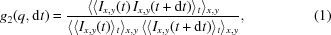where dt is the delay time, and Ix,y(t) and Ix,y(t+dt) are the scattering intensities at pixel (x,y) collected at times t and t+dt, respectively. The analysis of the g2 function allows one to determine the q-dependence of the characteristic time scale for the dynamics within the probed sample.

There are two types of analysis that are typically carried out dictated by the nature of the dynamics in the system which govern the functional form of dt referred to as the delay or the lag time. For a system exhibiting dynamics about equilibrium, dt can be described solely as a function of the difference in the time samples and is independent of any time origin. Thereby g2 can be described by a one-dimensional (1-D) correlation function as a function of dt at a given q. The most common algorithm that is used for this purpose is called the multi-tau' algorithm (Schatzel, 1990; Cipelletti & Weitz, 1999). It has been shown using extensive error analysis that g2 can be computed for logarithmic steps in dt instead of computing linearly spaced results at every possible value of dt. This results in significantly reduced computing resources without any smearing of the correlation function. A salient feature of this algorithm for the construction of g2 with logarithmic dt is the use of sampling time intervals that are increased in proportion to the delay time. This increase in sampling time causes a proportional increase in the signal per sampling interval and thus quickly reduces the photon noise contributions for large delay times dt and also aids in establishing a well defined baseline to g2 (Schatzel, 1990).

For systems exhibiting dynamics that are not in equilibrium, such as time-evolving systems where there is an origin of time, dt alone does not faithfully represent the dynamical behavior. The origin of time is specific to the system such as the onset of quenching during phase ordering (Fluerasu et al., 2005), and applied shear (Madsen et al., 2010; Rogers et al., 2014) to mention but a few. For describing the dynamical processes in such systems, the notion of a higher-order correlation function such as the two-time correlation function' was defined (Sutton et al., 2003). The two-time correlation function C(q,t1,t2) is defined as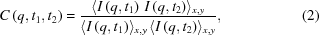where I(q,t1) and I(q,t2) are the intensities scattered at the momentum transfer q at time t1 and t2, respectively. The two-time correlation function provides a 2-D map of the dynamical phase space for all possible permutations and combinations of the times t1 and t2. The dynamical response of the system can be readily seen from such a map and further quantification of the correlations along different contours can be drawn, and is thus generally applicable to systems that are in non-equilibrium as well as in equilibrium. It can be readily seen that the computation of two-time correlations are far more compute intensive with a significantly increased memory footprint. It should be mentioned here for the sake of completion that the time delays are linearly spaced and the increase in sampling time for larger delays that was an integral part of the multi-tau algorithm is not applicable here and thereby requires a higher photon signal in each frame in the time series.

Fig. 2shows the different aspects of the computation results from a typical analysis using multi-tau and two-time algorithms. The results are described in detail in the caption.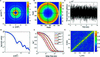Figure 2 Results of static and dynamic analysis from a colloidal suspension of 70 nm latex spheres dispersed in glycerol at a volume fraction of 1% measured with a Medixpix3-based LAMBDA detector are shown. The circular object is the 3 mm-diameter beam stop used to block the main beam from hitting the detector, and the horizontal and vertical bands are the dead regions between the modules in the detector array. (a) Time-averaged 2-D scattering pattern. (b) A pseudo color plot showing the digitized wavevector maps where each color corresponds to a mean wavevector value such that the range 0.02–0.2 nm−1 is divided into 140 linearly spaced wavevector bins. (c) Integrated scattering intensity over the user-specified area of interest plotted as a function of time which is typically used to assess for beam-induced damage to the sample. (d) Radially averaged small-angle X-ray scattering pattern as a function of wavevector. (e) Correlation functions (circles) along with simple exponential fits (solid lines) at wavevector values of 0.02, 0.03, 0.04 and 0.05 nm−1 in decreasing order of delay time. (f) Two-time correlation function computed at a wavevector value of 0.03 nm −1.

### 3. Parallel framework

A typical design pattern used in parallel programs is that of fork' and join', as shown in Fig. 3. In the fork phase, we spawn different instances of a program that run in parallel and work on different chunks of a problem, and then later the join phase combines individual outputs to form the final result. Our problem fits this design pattern; however, we are faced with handling a large volume of input and output data. The MapReduce (Dean & Ghemawat, 2008) framework solves this problem, as explained next, by extending the fork–join paradigm to a cluster of distributed-memory computers. Additionally, it offers a standard way of dealing with large volumes of data making it suitable for handling the complexity of our problem. In this section, we briefly explain the main features of MapReduce and the open source implementation of this framework, Hadoop.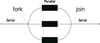Figure 3 A typical pattern found in the parallel implementation of many algorithms is the fork–join design pattern. The Hadoop MapReduce framework extends this idea to an HPC (high performance computing) cluster.

#### 3.1. MapReduce

MapReduce is a programming framework for processing large amounts of data using clusters of compute nodes. It was originally developed by Google for analyzing trillions of webpages for their search engine. The computation in MapReduce is divided into three phases: map, shuffle and reduce. The map phase splits the input data into smaller chunks called blocks. These blocks are processed in parallel by individual map processes. The output from each map process is a set of intermediate key-value pairs. Later, the shuffle phase groups these key-value pairs based on the value of each key such that all the values associated with a given key are available at a single reduce node. The reduce phase receives all the values associated with a single key as input from the shuffle process and produces the final output. Typically there is one map process per data block and one reduce process per key for maximum parallelism. The map and reduce functions are usually the only user-provided code; the rest of the heavy lifting of splitting the input into blocks, passing it to the maps and then sorting and combining the intermediate output and sending it to the appropriate reducer function is handled by the framework itself.

To illustrate the working of MapReduce, consider the example shown in Fig. 4that counts the occurrences of words in a document using MapReduce. For simplicity, we assume a single document containing a list of color names. The maps start by scanning parts of the input and produce an intermediate set of key-value pairs. The color name will become the key and the counts of the occurrence of that word within a block will be its value. During the shuffle phase the intermediate counts of the word occurrences are passed to the reducers. Each reducer is given counts for a single word. For example, our first reducer is passed the key w along with the list of counts from all the map operations. Each reducer then combines the counts of individual maps to form the final counts.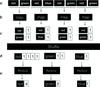Figure 4 A simple word count example to explain processing under the MapReduce framework. (a) The input is a series of words in a single document. (b) Each map scans part of the input. (c) An intermediate count of words is emitted by each map as a key-value pair. The word itself is the key and the count of its occurrence is the value. (d) Shuffle combines the intermediate key-values and passes them to reduce, where each reduce function obtains a distinct key along with all the intermediate values associated with that key. (e) Reduce computes the final count.

#### 3.2. Apache Hadoop

The MapReduce style of programming was further popularized by the free and open source implementation by the Apache Hadoop project (Apache, 2008). It provides a MapReduce framework that leverages a distributed file system (DFS) to make it easier to analyze large volumes of data. The DFS splits the files into blocks, referred to as splits in this text, of equal size and stores them across multiple machines in a cluster. The user submits their MapReduce code, called a job, following the semantics of the Application Programming Interface (API) provided by Hadoop. Upon invocation, Hadoop will launch the appropriate number of map and reduce processes depending on the capacity of the cluster, the size of the job and any other scheduling constraints. The number of mappers is usually equal to the number of data splits available for the given input file. The number of reducers is generally specified by the users and is typically set to the number of processing cores available for the job.

In addition to the basic filesystem and MapReduce capabilities, Hadoop offers many other desirable features including fault tolerance in the face of disk and machine failures, data and code co-locality that reduce latencies by moving the processing closer to the data, and the ability to run on commodity computer hardware, among many other features. The framework is written in the Java programming language and is available with different configuration and add-ons called distributions.

### 4. MapReduce for XPCS

In this section, we present our parallel implementation that utilizes the MapReduce paradigm to process XPCS data. The input to the system is image data collected by an area detector. The data coming from the detector are stored as a binary file where each frame is stored sequentially along with a per frame header containing the metadata information about that image. To make the discussion of the algorithm more concise we use the following notation to represent the input to the system. The input is represented as a series of N images where the superscripts indicate the frame number at a given time: [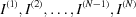]. An image I is a two-dimensional matrix of X rows and Y columns, where X and Y are the dimensions of the detector. The intensity of a single pixel at position x, y within a frame i is represented as Ix,y (i). Fig. 5shows a schematic view of our data.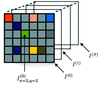Figure 5 I (i) is used to represent a single image in a sequence of frames. The notation I (i)x  =  j,y  =  k is used to refer to a specific pixel with x index j and y index k and frame number number i. The notation of x = X, y = Y refers to the last pixel in the image (lower right corner).

We have split the processing into two separate MapReduce jobs. The first job computes the auto-correlations for each pixel and stores the result in a binary file. The normalization of these per-pixel correlation values according to the user-specified length scale is performed by running an additional MapReduce job. In the section below, we explain the two MapReduce jobs. It should be mentioned here that, while the second MapReduce job could have been combined with the first, we implemented in two levels with no deterioration in the performance.

#### 4.1. Auto-correlations

Map. A set of map processes begin the analysis by processing individual data splits. The total number of maps is proportional to the total number of data splits which is equal to the block size. We use a block size of 128 MB. The framework tries to evenly allocate the data among the mappers. Each map processes its portion of the data split S containing some number of frames. The output of this phase is in the form of intermediate key-value pairs of the form [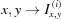]. Here, x and y are the positions of the pixels and the value part of this key, Ix,y (i) is the pixel intensity for that pixel along with its position in the frame sequence. It is important to note that at the map stage we are not computing any correlation. At this stage data are merely being re-organized, in parallel, for the reduce stage to compute per-pixel correlation using all frames in the data.

Reduce. Before launching the reduce processes, the framework combines all intermediate keys with a given value at a single compute node. In our case, all the intensity values for a given pixel position will be gathered and provided to a single reducer. In other words, a single reduce process is given a sequence of pixel intensities [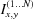] for a common pixel position x,y across all data frames. The reduce process then correlates the pixel intensities between different frames to compute the auto-correlation. There are two variations when deciding how the correlations should be done. These are multi-tau' or two-time' and are described next.

Multi-tau. In multi-tau, the correlation is computed such that the primary variable for controlling the time step is dt and it is typically set in logarithmic intervals as explained earlier. We compute the unnormalized correlations called G2 as a product of pixel values at frame i and frame i+dt as shown in equation (3),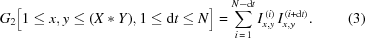Here, (X*Y) refers to the total number of pixels in the detector. One noteworthy point here is that equation (3)only shows a single iteration of the algorithm for any pixel. In multi-tau, the averaging of intensities is done at multiple levels. The number of levels depends on the number of frames and the number of time delays, dt, in each level, which typically has a default value of 8. Other than the first level, each level begins by first averaging out the pixel intensities in time from the previous level. In our implementation, we track correlations at each level using dt as part of the output key-value pair. All the computation for a single pixel, irrespective of the number of levels, is done by a single reduce process. This makes our implementation agnostic to the nature of the algorithm such as multi-tau or two-time.

Fig. 6summarizes the steps for computing auto-correlation using the multi-tau algorithm.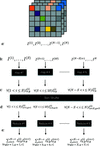Figure 6 (a) The area detector writes images as a series of frames. (b) The data are stored in a distributed filesystem where the data are split into blocks of equal size, S. These blocks are processed by individual maps. Each map handles a unique split of the data. (c) The pixel-intensity data per frame is emitted as intermediate data. (d) The framework combines pixel intensities for all frames for a given pixel and passes it to a reducer. (e) The reduce processes compute the auto-correlation of pixel-intensities across time.

Two-time. The two-time algorithm offers a slight variation to the multi-tau algorithm and is aimed towards characterizing the dynamics in non-equilibrium systems as explained earlier. Here, instead of the averaging of the frames that takes place in multi-tau in the higher levels resulting in dt being logarithmically spaced, correlation is carried out between every pair of frames in time. This results in a 2-D map of correlations, termed the two-time correlation matrix, with the time delay being linearly spaced. In comparison, the multi-tau algorithm results in a 1-D map of the correlations with the time delay being logarithmically spaced. Thereby, two-time calculations produce a large amount of output data. Equation (4)shows the actual calculation,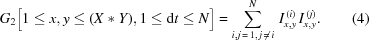The parallelization of two-time analysis follows roughly the same procedure as multi-tau. The data are split into blocks of small numbers of frames at the map stage. These sets of frames are processed (re-organized) in parallel and fed to the reduce processes. The reduce processes computes the co-relation for each pixel. However, instead of using a step function it computes a dot product of a one-dimensional pixel over the time array with itself.

#### 4.2. Normalization

The per pixel auto-correlations are then normalized based on the wavevector maps specified by the user. The wavevector maps are composed of bins such that each bin comprises a group of pixels based on the experimental geometry that are nominally equivalent and can therefore be normalized together. The normalization is carried out by running an additional MapReduce job that runs as soon as the pixel correlations are computed. The map' phase of that job reads the output from the previous job that consists of G2 values for each pixel for different time delays, dt. The emitted intermediate data from this map consists of the bin number of the pixel position and dt. The value for the intermediate key is the G2 correlation for the pixel at the given dt. These keys are then combined based on the bin number such that all the pixels that are in the same bin (wavevector) are available at a single reduce node. The reduce operation then averages them to compute normalized g2.

### 5. Performance

In this section, we present the performance of the MapReduce implementation using experimental datasets from the 8-ID-I beamline at the APS.

#### 5.1. Setup

Performance results are collected on a cluster of ten compute nodes with an additional node acting as head node. Both the compute and head nodes have two AMD Opteron 6220 processors with 12 cores each and 64 GB of memory. The cluster runs the Cloudera version CDH-5 (Cloudera, 2014) of the Hadoop file system and MapReduce. We measured the time it takes from submission to completion of our MapReduce jobs on the cluster. As there are no scheduling conflicts or multiple users competing for the cluster, a job typically starts immediately after submission.

We present the time it takes to complete MapReduce jobs for different sizes and numbers of frames. We also compare this time with the time it takes to collect these data with the LAMBDA detector operating at a frame rate of 2000 frames s−1 (Pennicard et al., 2011). This comparison is of particular interest as it sheds light on future efforts to bring the analysis time to within some small factor of the acquisition time to pave the way for real-time analysis.

We measured the time it takes to complete the analysis using both the multi-tau and two-time algorithms. Each frame consists of roughly one million pixels. About 20% of the pixels contain data while the remaining pixels are below the single-photon threshold and are removed by the areaDetector software (areaDetector, 2008) before writing the data to files. The data are stored as a sparse array with only those pixels stored that have a value above a threshold. Each pixel occupies 6 bytes: 4 bytes for the linear index of the pixel in the frame and 2 bytes for the intensity of the pixel.

#### 5.2. Results

The plot in Fig. 7shows the time it takes to complete the analysis using the multi-tau algorithm with a varying number of frames. We are able to process the majority of our data (ranging from 1 K to 8 K frames) in under 1 min. There is overhead in the MapReduce framework for setting up processes for maps, shufflers and reducers. This overhead dominates the overall time for a job when the number of frames are small. The time it takes to process up to 6000 frames is very similar for this reason. On the other hand, as we start increasing the frames, and thus the file size, the analysis time also starts to increase. This is due to the fact that most of the intermediate data produced for sorting and shuffling of key-value pairs is not going to fit in the memory and the MapReduce framework will have to start writing partial results to the disk. The extra reads and writes to and from the disk start to dominate the analysis time.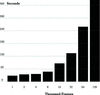Figure 7 Time (in seconds) to compute multi-tau correlations for data sets of different sizes.

The plot in Fig. 8compares the time it takes the MapReduce system to analyze the data with the time the acquisition system takes to acquire the data, which includes the data acquisition and data transfer to the analysis cluster. As one of our goals is to complete our analysis in near real-time, we are pushing for bringing these times as close to each other as possible. However, due to limitations of the MapReduce framework and its requirement of batch processing, our system still has overheads as shown in the plot.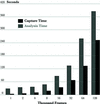Figure 8 Time (in seconds) to compute the multi-tau auto-correlation for a given data size in comparison with the time taken for collecting the same dataset. The analysis and capture time are shown side-by-side for better comparison.

The plot in Fig. 9is the analysis time using the two-time auto-correlation algorithm on data sets of different sizes. The amount of intermediate data produced by the two-time analysis is also much larger than that produced by the multi-tau algorithm. For each pixel the multi-tau algorithm produces a relatively smaller number of correlation values while the two-time calculations produces N correlations for each pixel. This difference also means that the amount of data to move within the cluster is usually higher for two-time. This is one of the reasons we restricted our analysis to <10000 frames for the two-time algorithm.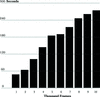Figure 9 Time (in seconds) to compute the two-time correlation for a given data size.

The plot in Fig. 10shows a comparison between analysis and acquisition time for the two-time correlations.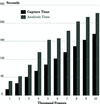Figure 10 Time comparison (in seconds) against number of frames for recording and analyzing data using the two-time auto-correlation algorithm. The analysis and capture times are stacked in the same plot for better comparison.

### 6. XPCS workflow using virtualized computing

In order to keep up with the demand for more computing resources, the APS has teamed up with the Computing, Environment and Life Sciences (CELS) directorate at Argonne to use Magellan, a virtualized computing resource. Virtual computing environments separate physical hardware resources from the software running on them, isolating an application from the physical platform. The use of this remote virtualized computing affords the APS many benefits. Magellan's virtualized environment allows the APS to install, configure and update its Hadoop-based XPCS reduction software easily and without interfering with other users on the system. Its scalability allows the APS to provision more computing resources when larger data sets are collected, and release those resources for others to use when not required. Further, the underlying hardware is supported and maintained by professional HPC engineers, relieving APS staff of this burden.

The XPCS workflow starts with raw data streaming directly from the detector, through an on-the-fly firmware discriminator to a compressed file on the parallel file system located at the APS. Once the acquisition is complete, the data are automatically transferred using GridFTP to the Hadoop Distributed File System (HDFS) running on the Magellan resource in the computing center located on the Argonne campus. This transfer occurs over two dedicated 10 Gb s−1 fiber optic links between the APS and the Magellan cluster. By bypassing intermediate firewalls, this dedicated connection provides a very low latency high-performance data pipe between the two facilities. Immediately after the transfer, the Hadoop MapReduce-based data reduction algorithms are run in parallel on the provisioned Magellan compute instances, followed by Python-based error-fitting code. Magellan resources provisioned for typical use by the XPCS application include approximately 120 CPU cores, 500 GB of distributed RAM, and 20 TB of distributed disk storage. Provenance information and the resultant reduced data are added to the original HDF5 file, which is automatically transferred back to the APS. Finally, the workflow pipeline triggers software for visualizing the data (see Fig. 11).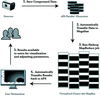Figure 11 The virtualized XPCS acquisition and analysis workflow system in use at the APS. These components are loosely connected via a well defined HDF5 file interface and a message-based workflow pipeline. (1) The detector data acquisition system writes data directly to a parallel file system located at the APS. (2) The workflow pipeline automatically transfers data from the APS to the Magellan resource over 2 × 10 Gb s−1 network links. (3) The Hadoop MapReduce-based autocorrelation job and subsequent fitting routines are run on the Magellan resource. (4) Reduced data are automatically transferred back to the APS. (5) The user views output visualizations and may adjust experiment parameters for subsequent acquisitions.

### 7. Conclusion and future work

We have presented a MapReduce-based implementation of computing auto-correlations for XPCS data at beamline 8-ID at the APS. Our system works on a cluster of computers and is able to process the majority of the datasets acquired at the beamline within a small factor of the acquisition time. This make it possible for our users to adjust experimental settings in near real-time and finish their analysis in a short span of time.

For the future, we note that the batch processing nature of Hadoop's MapReduce framework introduces additional delays. We are exploring other options to enable real-time streaming and reduction of our datasets including a parallel C++ solution using optimized math libraries and the Message Passing Interface (MPI) distributed framework.

### Acknowledgements

The work and the use of Advanced Photon Source is supported by US Department of Energy, Office of Science, under Contract No. DE-AC02-06CH11357. The authors thank Mitch McCuiston for the initiation of this project, Giampiero Sciutto for supporting the APS computing cluster, and Daniel Murphy-Olson and Ryan Aydelott for their support in setting up the Magellan virtualized environment.

### ReferencesJOURNAL OFSYNCHROTRONRADIATION